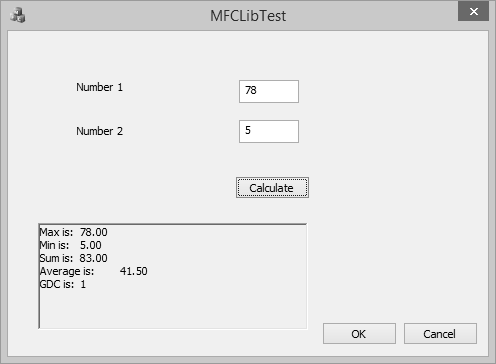# MFC - Libraries

A library is a group of functions, classes, or other resources that can be made available to programs that need already implemented entities without the need to know how these functions, classes, or resources were created or how they function. A library makes it easy for a programmer to use functions, classes, and resources etc. created by another person or company and trust that this external source is reliable and efficient. Some unique features related to libraries are −

• A library is created and functions like a normal regular program, using functions or other resources and communicating with other programs.

• To implement its functionality, a library contains functions that other programs would need to complete their functionality.

• At the same time, a library may use some functions that other programs would not need.

• The program that uses the library, are also called the clients of the library.

There are two types of functions you will create or include in your libraries −

• An internal function is one used only by the library itself and clients of the library will not need access to these functions.

• External functions are those that can be accessed by the clients of the library.

There are two broad categories of libraries you will deal with in your programs −

• Static libraries
• Dynamic libraries

## Static Library

A static library is a file that contains functions, classes, or resources that an external program can use to complement its functionality. To use a library, the programmer has to create a link to it. The project can be a console application, a Win32 or an MFC application. The library file has the lib extension.

Step 1 − Let us look into a simple example of static library by creating a new Win32 Project.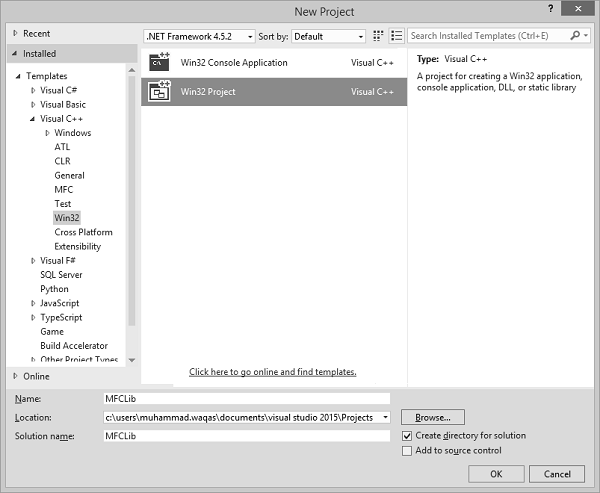Step 2 − On Application Wizard dialog box, choose the Static Library option.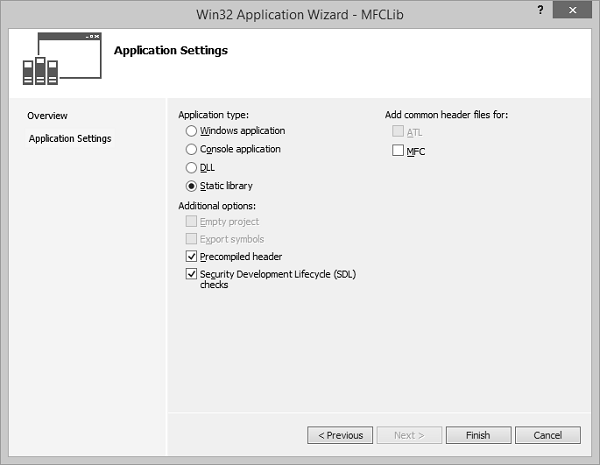Step 3 − Click Finish to continue.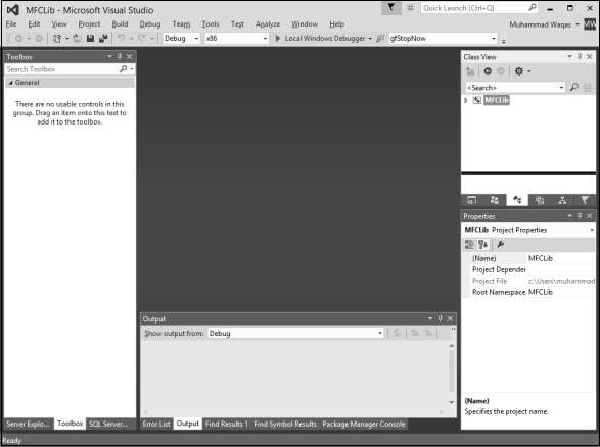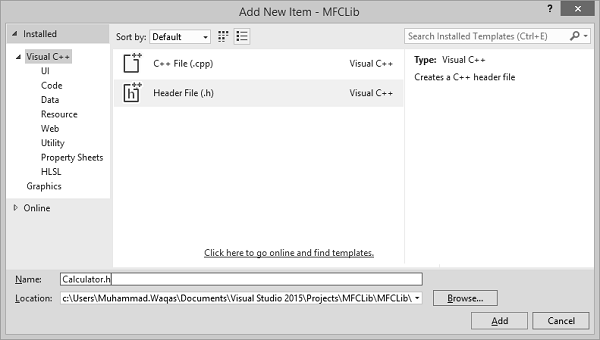Step 5 − Enter Calculator.h in the Name field and click Add.

```#pragma once
#ifndef _CALCULATOR_H_
#define _CALCULATOR_H_
double Min(const double *Numbers, const int Count);
double Max(const double *Numbers, const int Count);
double Sum(const double *Numbers, const int Count);
double Average(const double *Numbers, const int Count);
long GreatestCommonDivisor(long Nbr1, long Nbr2);
#endif // _CALCULATOR_H_
```

Step 6 − Add a source (*.cpp) file in the project.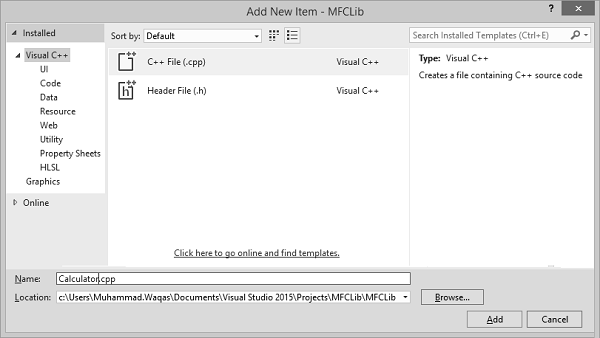Step 7 − Enter Calculator.cpp in the Name field and click Add.

Step 8 − Add the following code in the *.cpp file −

```#include "StdAfx.h"
#include "Calculator.h"
double Min(const double *Nbr, const int Total) {
double Minimum = Nbr;
for (int i = 0; i < Total; i++)
if (Minimum > Nbr[i])
Minimum = Nbr[i];
return Minimum;
}
double Max(const double *Nbr, const int Total) {
double Maximum = Nbr;
for (int i = 0; i < Total; i++)
if (Maximum < Nbr[i])
Maximum = Nbr[i];
return Maximum;
}
double Sum(const double *Nbr, const int Total) {
double S = 0;
for (int i = 0; i < Total; i++)
S += Nbr[i];
return S;
}
double Average(const double *Nbr, const int Total) {
double avg, S = 0;
for (int i = 0; i < Total; i++)
S += Nbr[i];
avg = S / Total;
return avg;
}
long GreatestCommonDivisor(long Nbr1, long Nbr2) {
while (true) {
Nbr1 = Nbr1 % Nbr2;
if (Nbr1 == 0)
return Nbr2;
Nbr2 = Nbr2 % Nbr1;
if (Nbr2 == 0)
return Nbr1;
}
}
```

Step 9 − Build this library from the main menu, by clicking Build → Build MFCLib.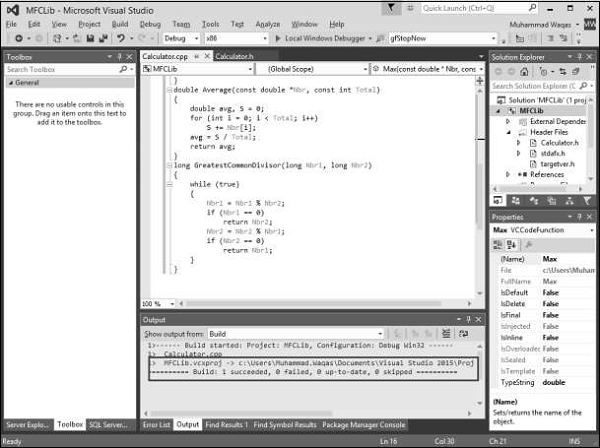Step 10 − When library is built successfully, it will display the above message.

Step 11 − To use these functions from the library, let us add another MFC dialog application based from File → New → Project.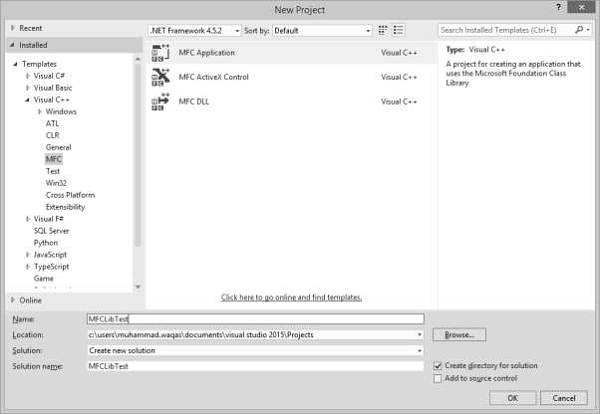Step 12 − Go to the MFCLib\Debug folder and copy the header file and *.lib files to the MFCLibTest project as shown in the following snapshot.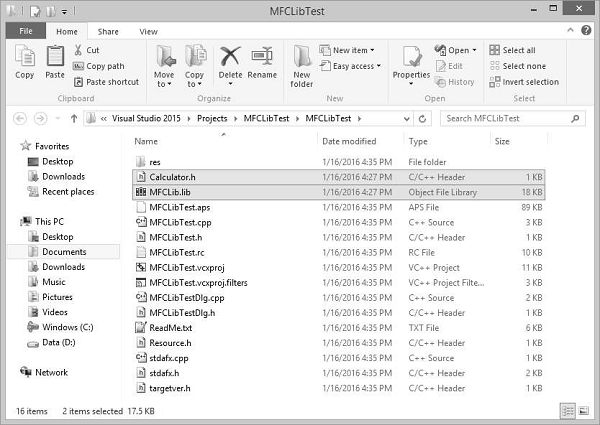Step 13 − To add the library to the current project, on the main menu, click Project → Add Existing Item and select MFCLib.lib.

Step 14 − Design your dialog box as shown in the following snapshot.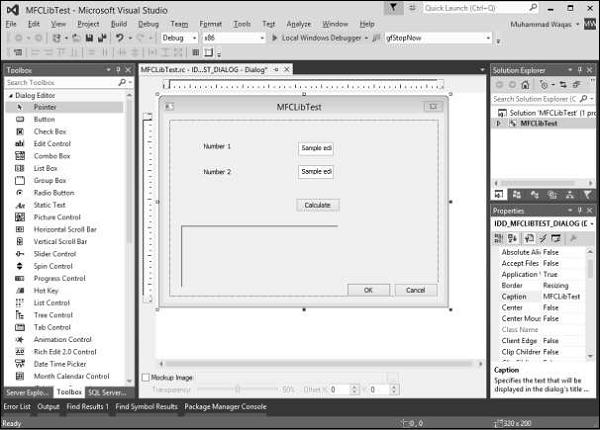Step 15 − Add value variable for both edit controls of value type double.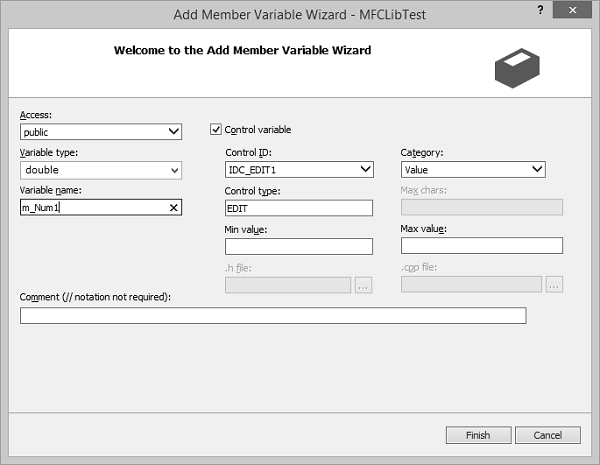Step 16 − Add value variable for Static text control, which is at the end of the dialog box.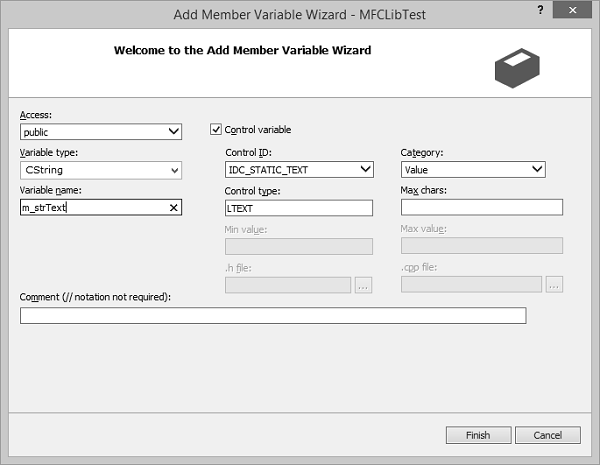Step 17 − Add the event handler for Calculate button.

To add functionality from the library, we need to include the header file in CMFCLibTestDlg.cpp file.

```#include "stdafx.h"
#include "MFCLibTest.h"
#include "MFCLibTestDlg.h"
#include "afxdialogex.h"
#include "Calculator.h"
```

Step 18 − Here is the implementation of button event handler.

```void CMFCLibTestDlg::OnBnClickedButtonCal() {
UpdateData(TRUE);
CString strTemp;
double numbers;
numbers = m_Num1;
numbers = m_Num2;

strTemp.Format(L"%.2f", Max(numbers,2));
m_strText.Append(L"Max is:\t" + strTemp);

strTemp.Format(L"%.2f", Min(numbers, 2));
m_strText.Append(L"\nMin is:\t" + strTemp);

strTemp.Format(L"%.2f", Sum(numbers, 2));
m_strText.Append(L"\nSum is:\t" + strTemp);

strTemp.Format(L"%.2f", Average(numbers, 2));
m_strText.Append(L"\nAverage is:\t" + strTemp);

strTemp.Format(L"%d", GreatestCommonDivisor(m_Num1, m_Num2));
m_strText.Append(L"\nGDC is:\t" + strTemp);

UpdateData(FALSE);
}
```

Step 19 − When the above code is compiled and executed, you will see the following output.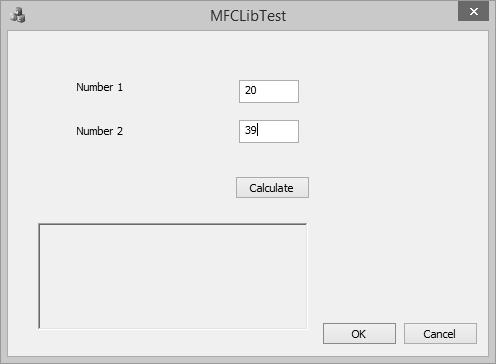Step 20 − Enter two values in the edit field and click Calculate. You will now see the result after calculating from the library.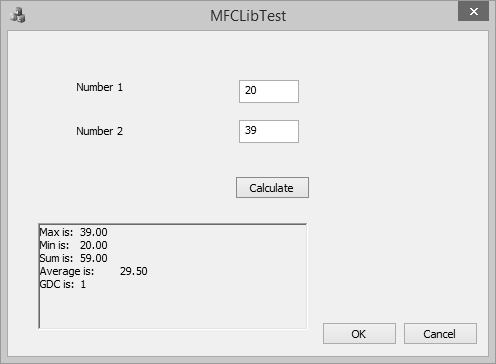## Dynamic Library

A Win32 DLL is a library that can be made available to programs that run on a Microsoft Windows computer. As a normal library, it is made of functions and/or other resources grouped in a file.

The DLL abbreviation stands for Dynamic Link Library. This means that, as opposed to a static library, a DLL allows the programmer to decide on when and how other applications will be linked to this type of library.

For example, a DLL allows difference applications to use its library as they see fit and as necessary. In fact, applications created on different programming environments can use functions or resources stored in one particular DLL. For this reason, an application dynamically links to the library.

Step 1 − Let us look into a simple example by creating a new Win32 Project.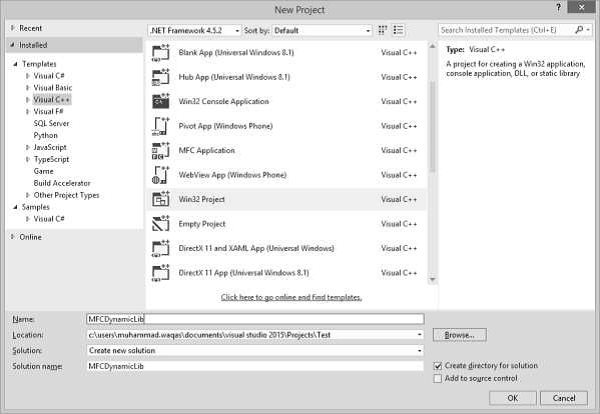Step 2 − In the Application Type section, click the DLL radio button.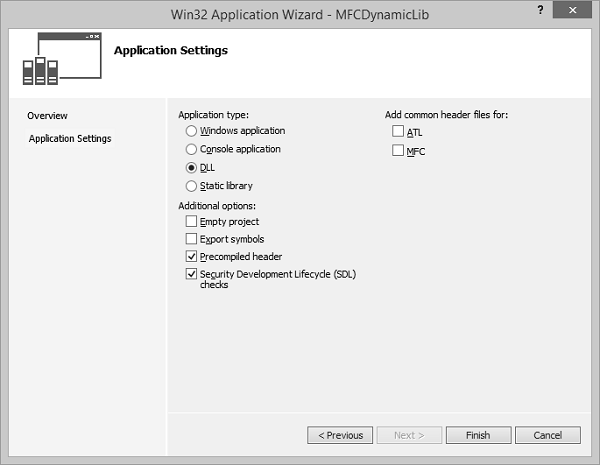Step 3 − Click Finish to continue.

Step 4 − Add the following functions in MFCDynamicLib.cpp file and expose its definitions by using −

```extern "C" _declspec(dllexport)
```

Step 5 − Use the _declspec(dllexport) modifier for each function that will be accessed outside the DLL.

```// MFCDynamicLib.cpp : Defines the exported functions for the DLL application.//

#include "stdafx.h"

extern "C" _declspec(dllexport) double Min(const double *Numbers, const int Count);
extern "C" _declspec(dllexport) double Max(const double *Numbers, const int Count);
extern "C" _declspec(dllexport) double Sum(const double *Numbers, const int Count);
extern "C" _declspec(dllexport) double Average(const double *Numbers, const int Count);
extern "C" _declspec(dllexport) long GreatestCommonDivisor(long Nbr1, long Nbr2);

double Min(const double *Nbr, const int Total) {
double Minimum = Nbr;
for (int i = 0; i < Total; i++)
if (Minimum > Nbr[i])
Minimum = Nbr[i];
return Minimum;
}
double Max(const double *Nbr, const int Total) {
double Maximum = Nbr;
for (int i = 0; i < Total; i++)
if (Maximum < Nbr[i])
Maximum = Nbr[i];
return Maximum;
}
double Sum(const double *Nbr, const int Total) {
double S = 0;
for (int i = 0; i < Total; i++)
S += Nbr[i];
return S;
}
double Average(const double *Nbr, const int Total){
double avg, S = 0;
for (int i = 0; i < Total; i++)
S += Nbr[i];
avg = S / Total;
return avg;
}
long GreatestCommonDivisor(long Nbr1, long Nbr2) {
while (true) {
Nbr1 = Nbr1 % Nbr2;
if (Nbr1 == 0)
return Nbr2;
Nbr2 = Nbr2 % Nbr1;
if (Nbr2 == 0)
return Nbr1;
}
}
```

Step 6 − To create the DLL, on the main menu, click Build > Build MFCDynamicLib from the main menu.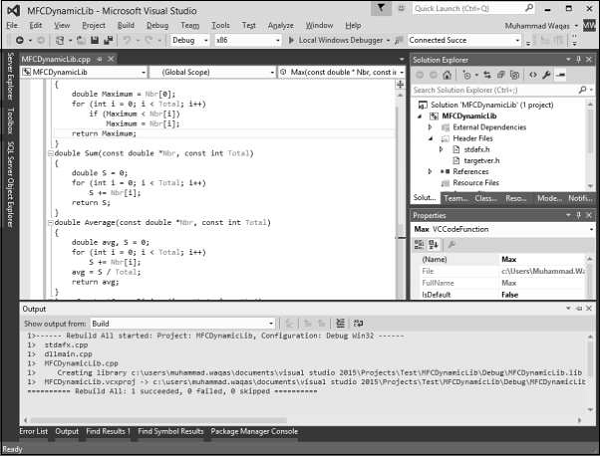Step 7 − Once the DLL is successfully created, you will see amessage display in output window.

Step 8 − Open Windows Explorer and then the Debug folder of the current project.

Step 9 − Notice that a file with dll extension and another file with lib extension has been created.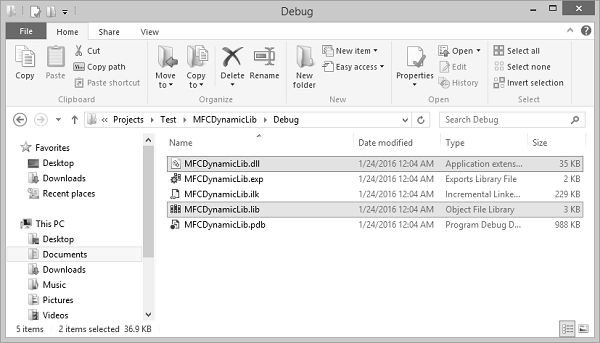Step 10 − To test this file with dll extension, we need to create a new MFC dialog based application from File → New → Project.Step 11 − Go to the MFCDynamicLib\Debug folder and copy the *.dll and *.lib files to the MFCLibTest project as shown in the following snapshot.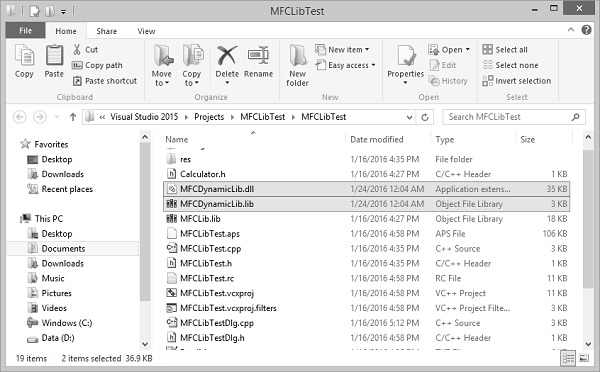Step 12 − To add the DLL to the current project, on the main menu, click Project → Add Existing Item and then, select MFCDynamicLib.lib file.

Step 13 − Design your dialog box as shown in the following snapshot.Step 14 − Add value variable for both edit controls of value type double.Step 15 − Add value variable for Static text control, which is at the end of the dialog box.Step 16 − Add the event handler for Calculate button.

Step 17 − In the project that is using the DLL, each function that will be accessed must be declared using the _declspec(dllimport) modifier.

Step 18 − Add the following function declaration in MFCLibTestDlg.cpp file.

```extern "C" _declspec(dllimport) double Min(const double *Numbers, const int Count);
extern "C" _declspec(dllimport) double Max(const double *Numbers, const int Count);
extern "C" _declspec(dllimport) double Sum(const double *Numbers, const int Count);
extern "C" _declspec(dllimport) double Average(const double *Numbers, const int Count);
extern "C" _declspec(dllimport) long GreatestCommonDivisor(long Nbr1, long Nbr2);
```

Step 19 − Here is the implementation of button event handler.

```void CMFCLibTestDlg::OnBnClickedButtonCal() {

UpdateData(TRUE);

CString strTemp;
double numbers;
numbers = m_Num1;
numbers = m_Num2;

strTemp.Format(L"%.2f", Max(numbers,2));
m_strText.Append(L"Max is:\t" + strTemp);

strTemp.Format(L"%.2f", Min(numbers, 2));
m_strText.Append(L"\nMin is:\t" + strTemp);

strTemp.Format(L"%.2f", Sum(numbers, 2));
m_strText.Append(L"\nSum is:\t" + strTemp);

strTemp.Format(L"%.2f", Average(numbers, 2));
m_strText.Append(L"\nAverage is:\t" + strTemp);

strTemp.Format(L"%d", GreatestCommonDivisor(m_Num1, m_Num2));
m_strText.Append(L"\nGDC is:\t" + strTemp);

UpdateData(FALSE);
}
```

Step 20 − When the above code is compiled and executed, you will see the following output.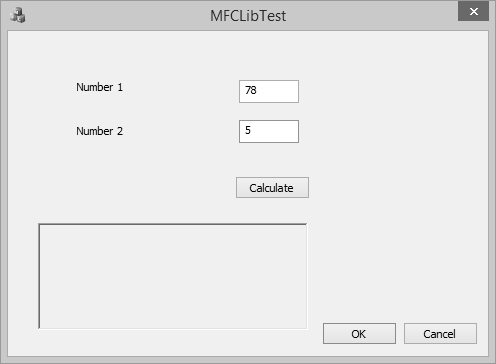Step 21 − Enter two values in the edit field and click Calculate. You will now see the result after calculating from the DLL.